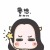0+0+0+0+0+0+0+0+0+0+0+0+Dynamic Time Warping (DTW) is a well-known similarity measure for time series. The standard dynamic programming approach to compute the DTW distance of two length-\$n\$ time series, however, requires \$O(n^2)\$ time, which is often too slow for real-world applications. Therefore, many heuristics have been proposed to speed up the DTW computation. These are often based on approximating or bounding the true DTW distance or considering special input data (e.g. binary or piecewise constant time series). In this paper, we present an exact algorithm to compute the DTW distance of two run-length encoded time series. The worst-case running time is cubic in the encoding length. In practice, however, our algorithm might be faster and used for accurate indexing and classification of time series in combination with preprocessing techniques such as piecewise aggregate approximation (PAA).0+0+0+下载预览Dimensionality reduction is a critical step in scaling machine learning pipelines. Principal component analysis (PCA) is a standard tool for dimensionality reduction, but performing PCA over a full dataset can be prohibitively expensive. As a result, theoretical work has studied the effectiveness of iterative, stochastic PCA methods that operate over data samples. However, termination conditions for stochastic PCA either execute for a predetermined number of iterations, or until convergence of the solution, frequently sampling too many or too few datapoints for end-to-end runtime improvements. We show how accounting for downstream analytics operations during DR via PCA allows stochastic methods to efficiently terminate after operating over small (e.g., 1%) subsamples of input data, reducing whole workload runtime. Leveraging this, we propose DROP, a DR optimizer that enables speedups of up to 5x over Singular-Value-Decomposition-based PCA techniques, and exceeds conventional approaches like FFT and PAA by up to 16x in end-to-end workloads.0+0+0+下载预览0+0+0+Dynamic Time Warping (DTW) is a well-known similarity measure for time series. The standard dynamic programming approach to compute the DTW distance of two length-\$n\$ time series, however, requires \$O(n^2)\$ time, which is often too slow for real-world applications. Therefore, many heuristics have been proposed to speed up the DTW computation. These are often based on approximating or bounding the true DTW distance or considering special input data (e.g. binary or piecewise constant time series). In this paper, we present an exact algorithm to compute the DTW distance of two run-length encoded time series. The worst-case running time is cubic in the encoding length. In practice, however, our algorithm might be faster and used for accurate indexing and classification of time series in combination with preprocessing techniques such as piecewise aggregate approximation (PAA).0+0+0+下载预览Symbolic Aggregate approximation (SAX) is a classical symbolic approach in many time series data mining applications. However, SAX only reflects the segment mean value feature and misses important information in a segment, namely the trend of the value change in the segment. Such a miss may cause a wrong classification in some cases, since the SAX representation cannot distinguish different time series with similar average values but different trends. In this paper, we present Trend Feature Symbolic Aggregate approximation (TFSAX) to solve this problem. First, we utilize Piecewise Aggregate Approximation (PAA) approach to reduce dimensionality and discretize the mean value of each segment by SAX. Second, extract trend feature in each segment by using trend distance factor and trend shape factor. Then, design multi-resolution symbolic mapping rules to discretize trend information into symbols. We also propose a modified distance measure by integrating the SAX distance with a weighted trend distance. We show that our distance measure has a tighter lower bound to the Euclidean distance than that of the original SAX. The experimental results on diverse time series data sets demonstrate that our proposed representation significantly outperforms the original SAX representation and an improved SAX representation for classification.0+0+0+下载预览0+0+0+0+0+0+Dynamic Time Warping (DTW) is a well-known similarity measure for time series. The standard dynamic programming approach to compute the dtw-distance of two length-\$n\$ time series, however, requires \$O(n^2)\$ time, which is often too slow in applications. Therefore, many heuristics have been proposed to speed up the dtw computation. These are often based on approximating or bounding the true dtw-distance or considering special inputs (e.g. binary or piecewise constant time series). In this paper, we present a fast and exact algorithm to compute the dtw-distance of two run-length encoded time series. This might be used for fast and accurate indexing and classification of time series in combination with preprocessing techniques such as piecewise aggregate approximation (PAA).0+0+0+下载预览0+0+0+0+0+0+0+0+0+
Top